Examples

Chapter 3 Class 11 Trigonometric Functions
Serial order wise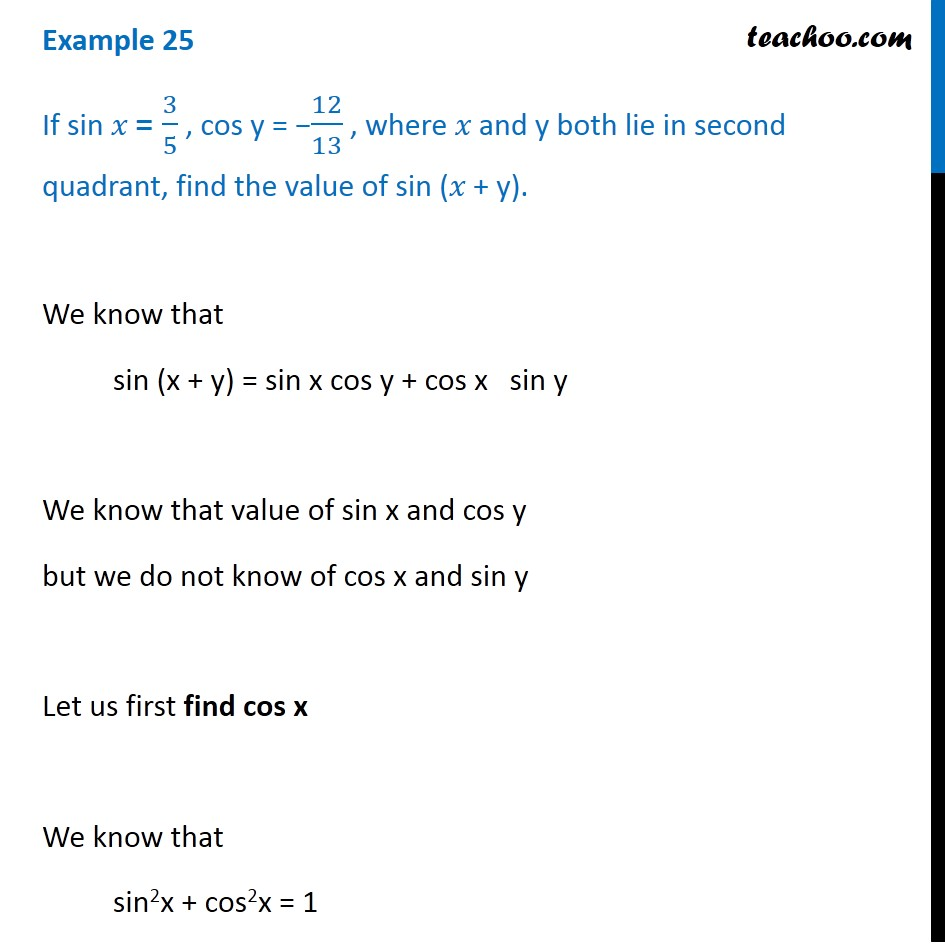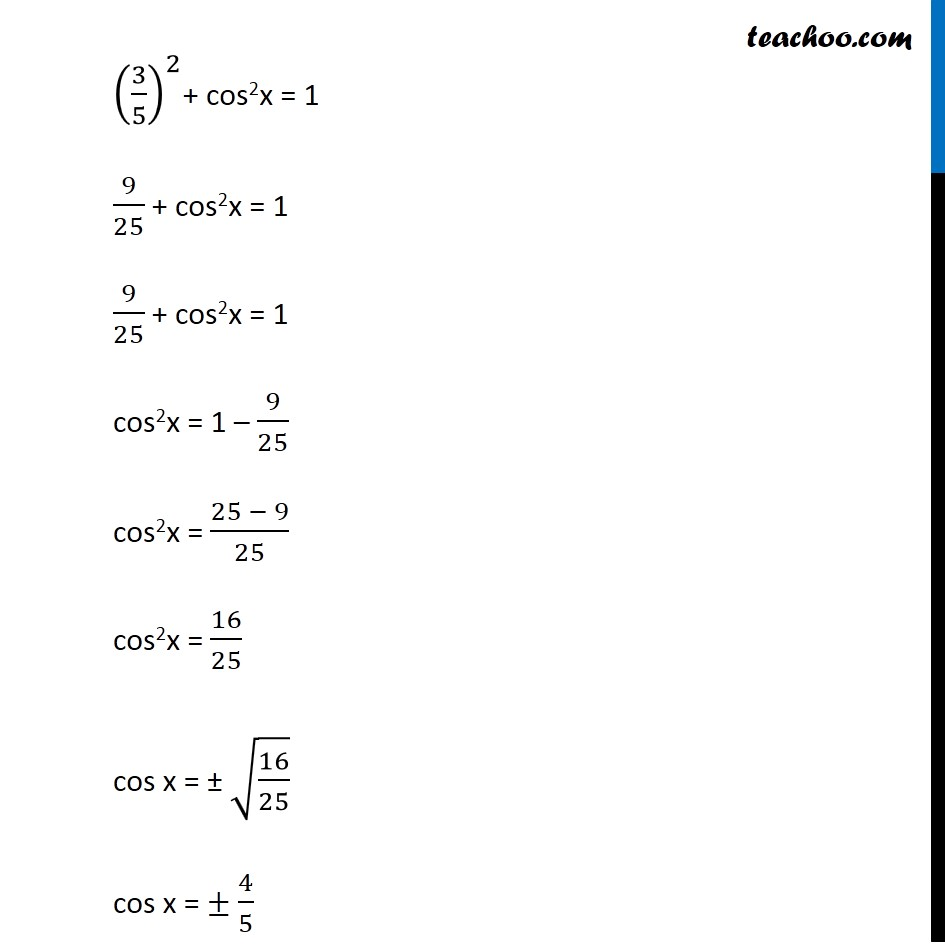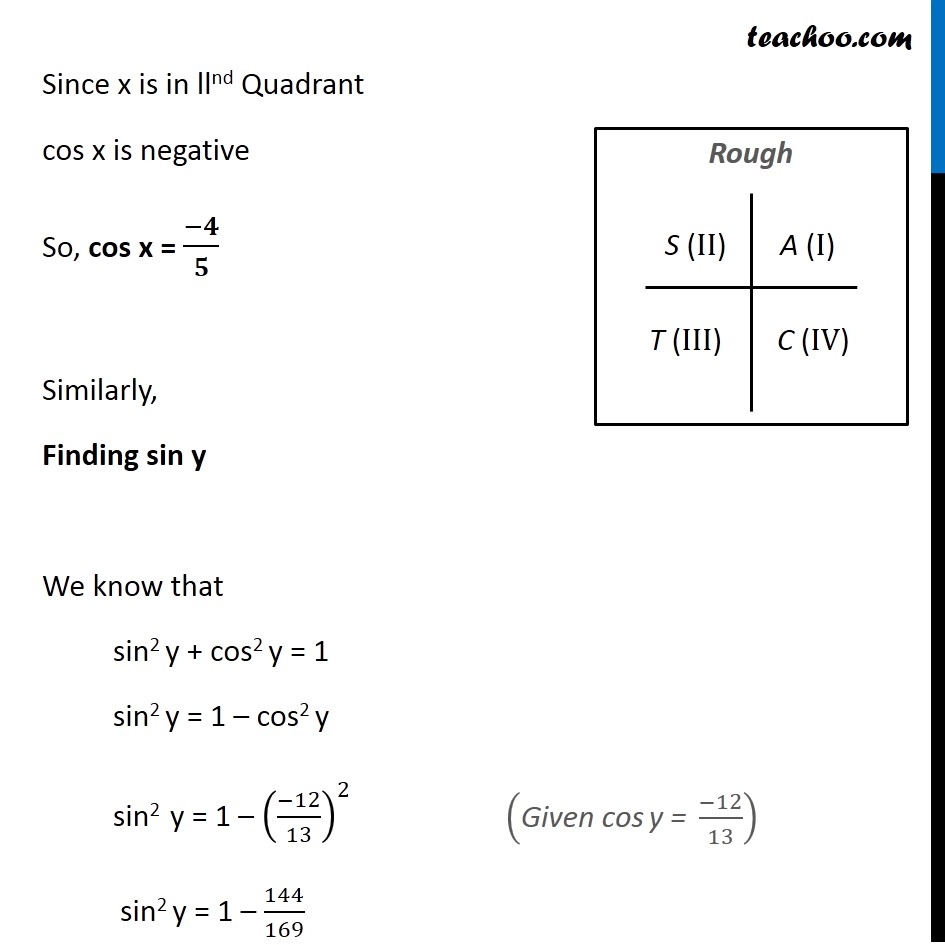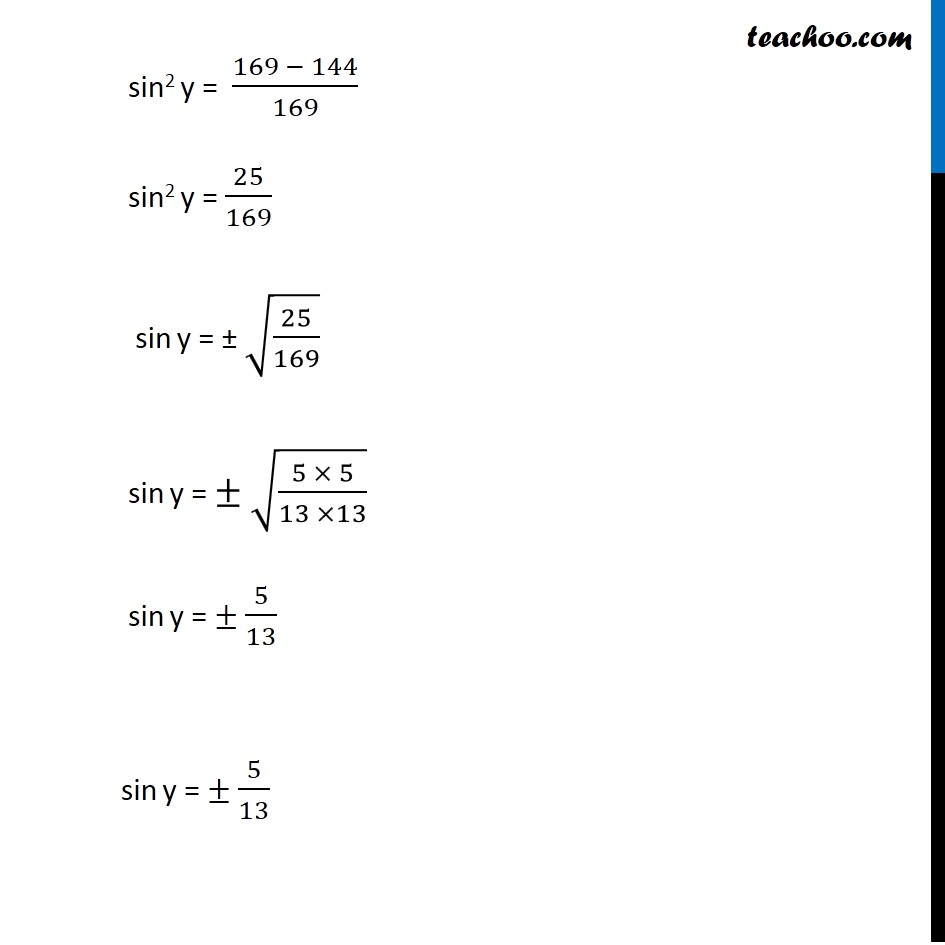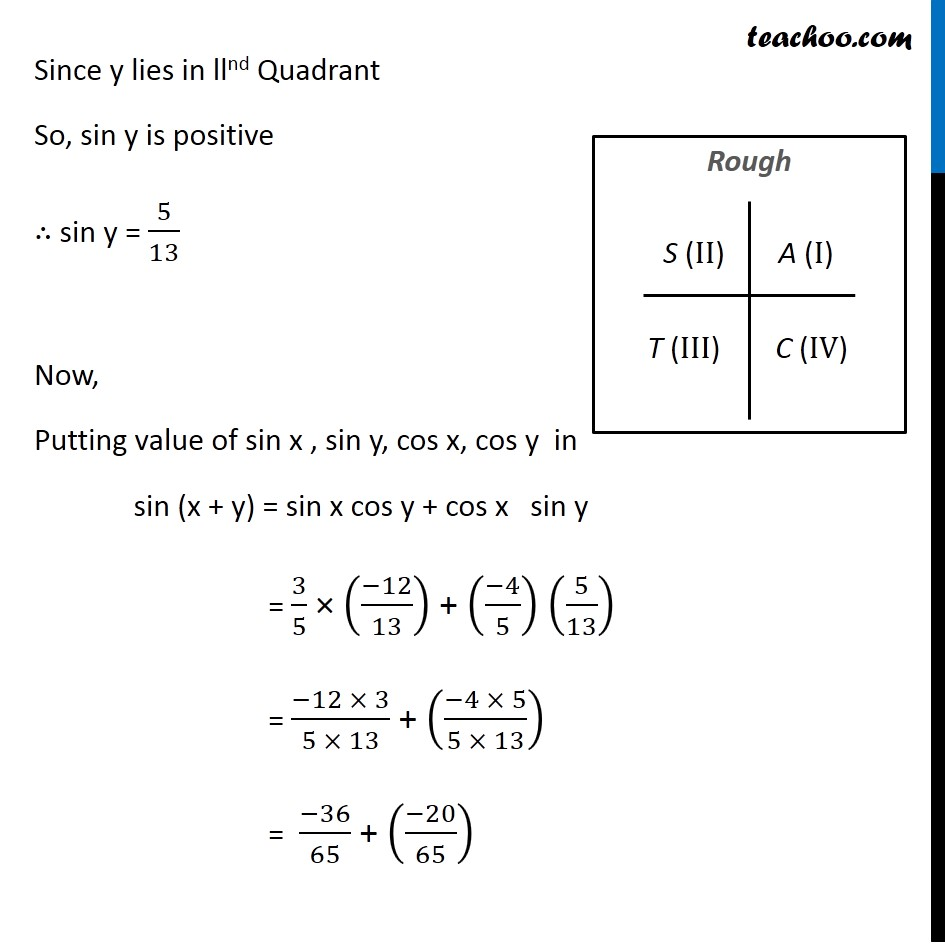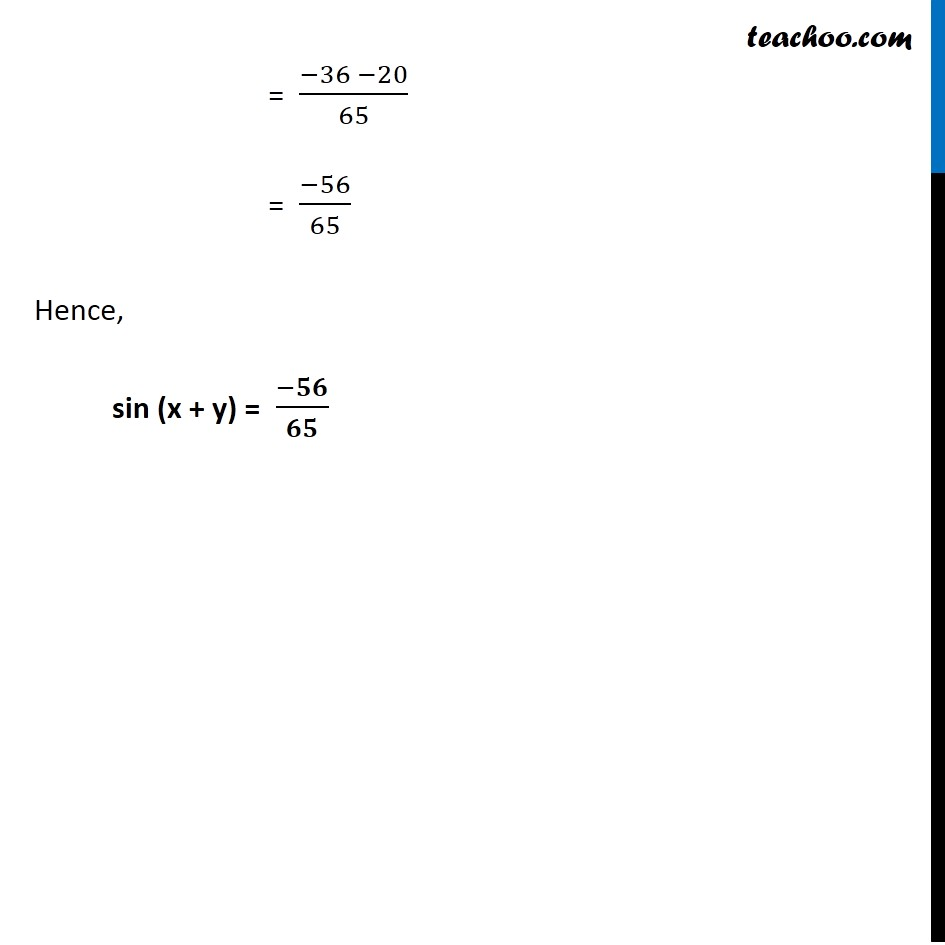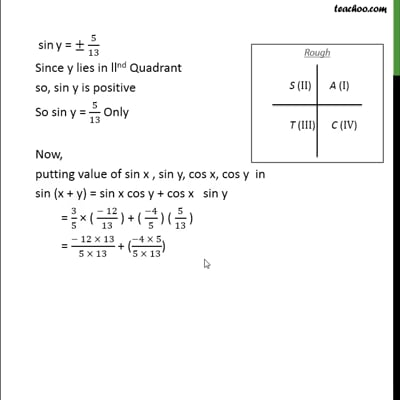This video is only available for Teachoo black users

Maths Crash Course - Live lectures + all videos + Real time Doubt solving!

### Transcript

Example 25 If sin 𝑥 = 3/5 , cos y = −12/13 , where 𝑥 and y both lie in second quadrant, find the value of sin (𝑥 + y). We know that sin (x + y) = sin x cos y + cos x sin y We know that value of sin x and cos y but we do not know of cos x and sin y Let us first find cos x We know that sin2x + cos2x = 1 (3/5)^2+ cos2x = 1 9/25 + cos2x = 1 9/25 + cos2x = 1 cos2x = 1 – 9/25 cos2x = (25 − 9)/25 cos2x = 16/25 cos x = ± √(16/25) cos x = ± 4/5 Since x is in llnd Quadrant cos x is negative So, cos x = (−𝟒)/𝟓 Similarly, Finding sin y We know that sin2 y + cos2 y = 1 sin2 y = 1 – cos2 y sin2 y = 1 – ((−12)/13)^2 sin2 y = 1 – 144/169 ("Given cos y =" (−12)/13) sin2 y = (169 − 144)/169 sin2 y = 25/169 sin y = ± √(25/169) sin y = ± √((5 × 5)/(13 ×13)) sin y = ± 5/13 sin y = ± 5/13 Since y lies in llnd Quadrant So, sin y is positive ∴ sin y = 5/13 Now, Putting value of sin x , sin y, cos x, cos y in sin (x + y) = sin x cos y + cos x sin y = 3/5 × ((−12)/13) + ((−4)/5) (5/13) = (−12 × 3)/(5 × 13) + ((−4 × 5)/(5 × 13)) = (−36)/65 + ((−20)/65) = (−36 −20)/65 = (−56)/65 Hence, sin (x + y) = (−𝟓𝟔)/𝟔𝟓